# Water/Mirror Image Questions For SSC CGL PDF

0
9686

Water/Mirror Image Questions For SSC CGL PDF:’

Download PDF of Image refelction Questions & Answers for SSC CGL exam based on non verbal asked questions in SSC exams with solutions.

Question 1: Which of the following is the water reflection of the given image?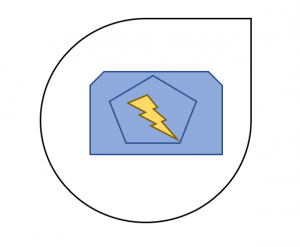a)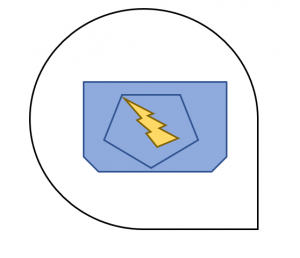b)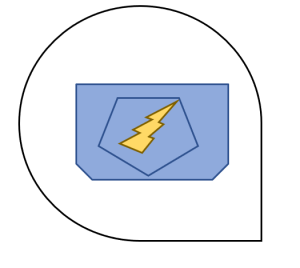c)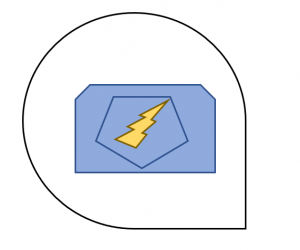d)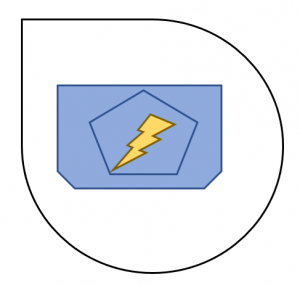Question 2: Which of the following is the water reflection of the given image?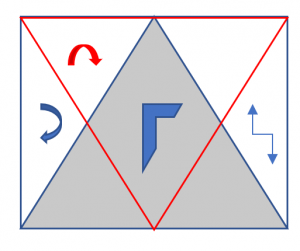a)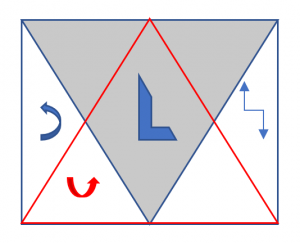b)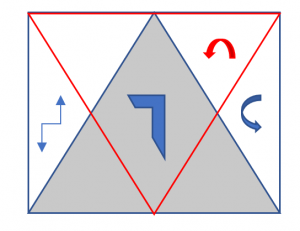c)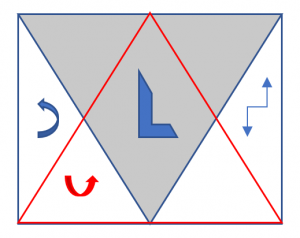d)Question 3: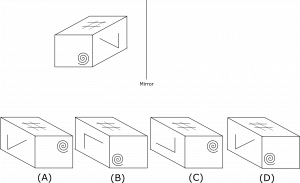Question 4: Which of the following is the mirror image of the figure given.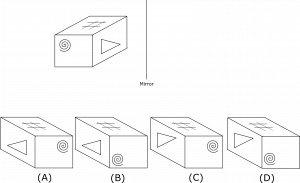Question 5: In the following question, four figures are given. Out of the four, three figures are similar while the remaining one is odd. Find the odd figure.

a)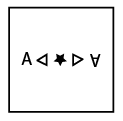b)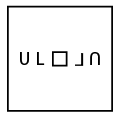c)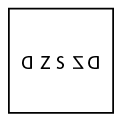d)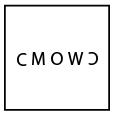Option B is the water reflection of the given image.
In option A the ‘lightning’ symbol is incorrectly placed.
In option C the ‘lightning’ symbol is incorrectly placed.
In option D the outer symbol is not the water reflection of the given outer image.

Option C is the water reflection of the given figure.
Option B is the mirror reflection.
Option D is the same figure.
In Option A the arrows are not pointing correctly.

The spiral is on the left bottom of the front face of the figure as shown.
In the mirror reflection it should be in the right bottom. This is followed only in the options B and D.
Thus options A and C can be eliminated.
In option B the reflection of 7 changed into the shape of L.
This is not possible as the angle between the line segments cannot change due to reflection.
Thus option B can also be eliminated.
Option D is the correct mirror image of the given figure and is thus the answer.